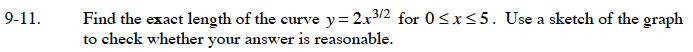### Home > CALC > Chapter 9 > Lesson 9.1.1 > Problem9-11

9-11.

Find the exact length of the curve y = 2x3/2 for 0 ≤ x ≤ 5. Use a sketch of the graph to check whether your answer is reasonable. Homework Help ✎The general formula for arc length is:

$=\left.\Big(\frac{1}{9}\Big)\Big(\frac{2}{3}\Big)(1+9x)^{3/2}\right|_0^5$